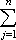#Interactive Real Analysis

Next | Previous | Glossary | Map

## 7.1. Riemann Integral

### Theorem 7.1.14: Riemann Integrals of Continuous Functions

Every continuous function on a closed, bounded interval is Riemann integrable. The converse is false.

### Proof:

We have shown before that f(x) = x2 is integrable where we used the fact that f was differentiable. We will now adjust that proof to this situation, using uniform continuity instead of differentiability. We can actually simplify the previous proof because we now have Riemann's lemma at our disposal.

We know that f is continuous over a closed and bounded interval. Therefore f must be uniformly continuous over [a, b], i.e. for any given> 0 we can find a> 0 such that |f(x) - f(y)| <for all x and y with |x - y| <.

Now take any> 0 and choose a partition P with |P| <. Then

| U(f, P) - L(f, P)||cj - dj| (xj - xj-1)
where cj is the sup of f over [xj-1, xj] and dj is the inf over that interval. Since the function is continuous, it assumes its maximum and minimum over each of the subintervals, so that inf and sup can be replaced with min and max.

Since the norm of the partition is less thanwe know that |cj - dj| <for all j.

But then

| U(f, P) - L(f, P)|(xj - xj-1)
=(xj - xj-1)
=(xn - x0)
=(b - a)
because the last sum is telescoping. That finishes the proof.

For the purist, we should have chosensuch that |f(x) - f(y)| </ (b-a) whenever |x - y| <so that at the end of our above computation we could get a single. But that's just details...

It is easy to find an example of a function that is Riemann integrable but not continuous. For example, the function f that is equal to -1 over the interval [0, 1] and +1 over the interval [1, 2] is not continuous but Riemann integrable (show it!).Next | Previous | Glossary | Map